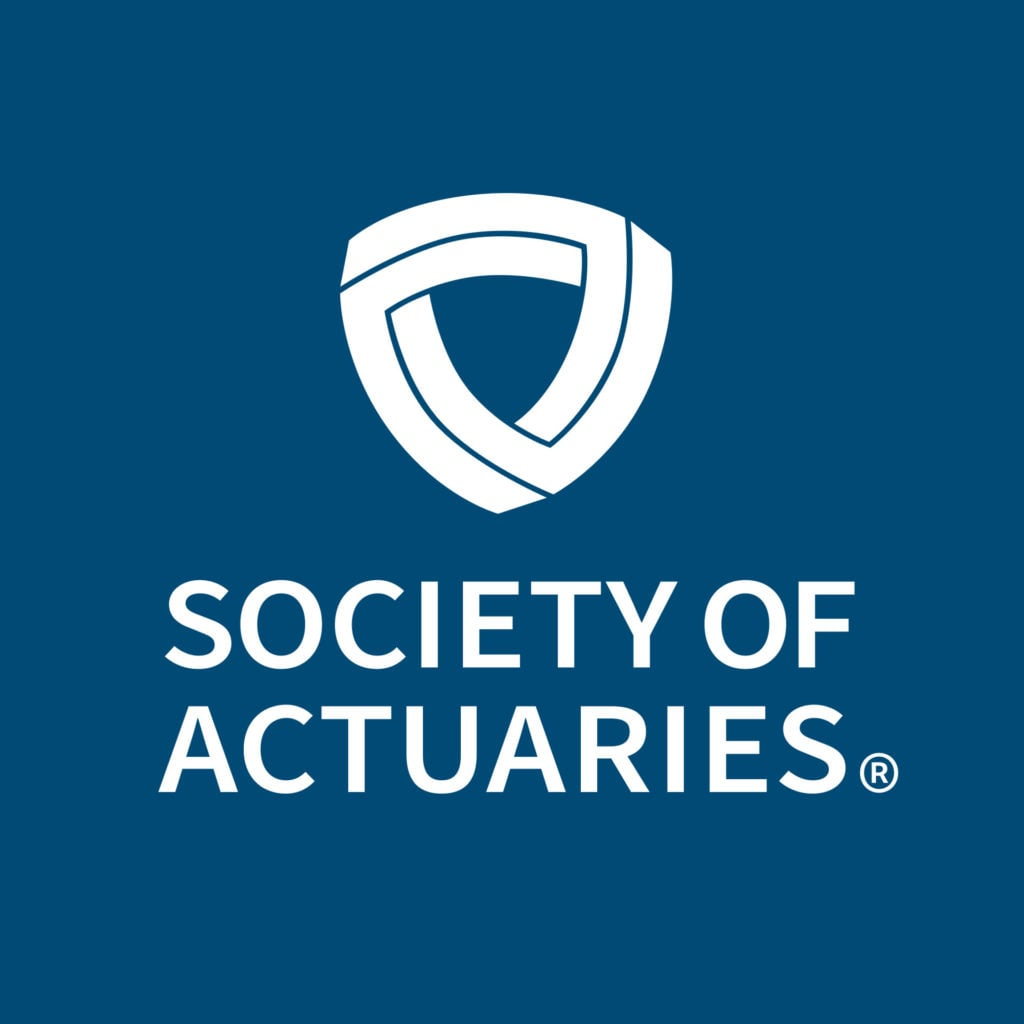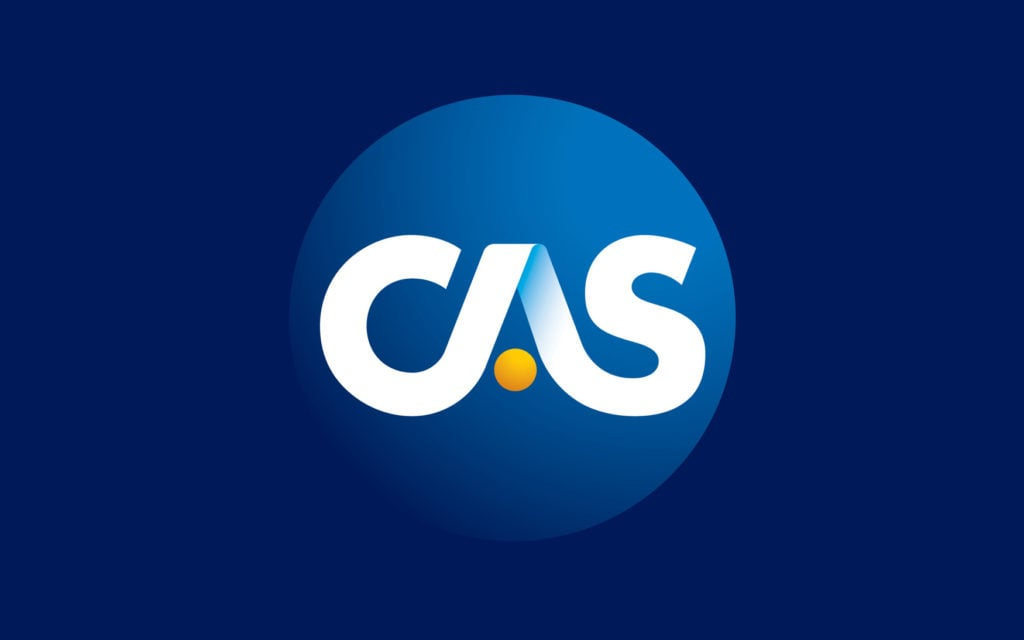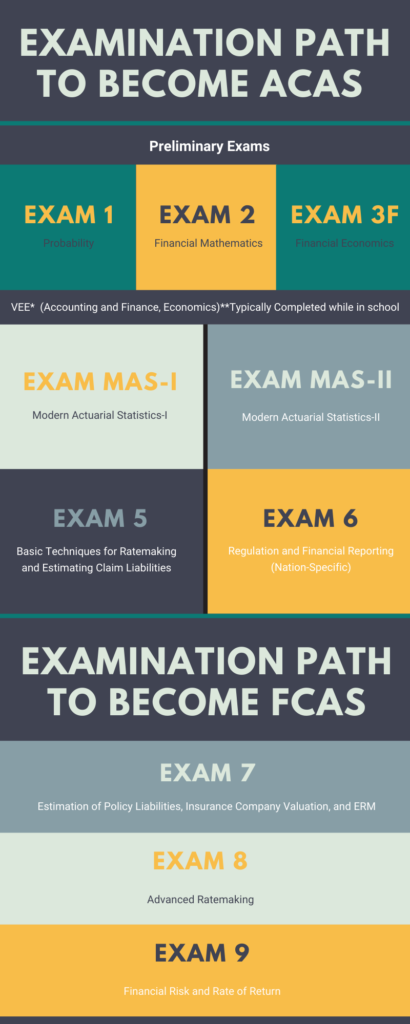# Exams

## Preliminary Exams

Probability Exam (SOA Exam P / CAS Exam 1): The exam consists of three hours of multiple-choice questions. The purpose of this exam is to develop knowledge of probability. The application of these tools to problems encountered by actuaries is emphasized. A thorough command of calculus and probability topics is assumed. Additionally, a very basic knowledge of insurance and risk management is assumed.

Financial Mathematics Exam (SOA Exam FM / CAS Exam 2): The exam consists of three hours of multiple-choice questions. This exam covers interest theory (discrete and continuous) and an introduction to derivative securities. It assumes a basic knowledge of calculus and probability.

Investment and Financial Markets (SOA Exam IFM / CAS Exam 3F): The exam consists of three hours of multiple-choice questions. The syllabus for Exam IFM develops the candidate’s knowledge of the theoretical basis of corporate finance and financial models and the application of those models to insurance and other financial risks. A thorough knowledge of calculus, probability (as covered in Exam P), basic corporate finance (as covered in VEE Accounting and Corporate Finance) and interest theory (as covered in Exam FM) is assumed.## CAS Exams